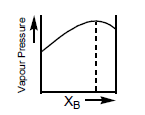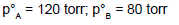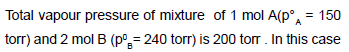Courses

# Solutions (Chapter Test - Medical)

## 20 Questions MCQ Test | Solutions (Chapter Test - Medical)

Description
This mock test of Solutions (Chapter Test - Medical) for Class 12 helps you for every Class 12 entrance exam. This contains 20 Multiple Choice Questions for Class 12 Solutions (Chapter Test - Medical) (mcq) to study with solutions a complete question bank. The solved questions answers in this Solutions (Chapter Test - Medical) quiz give you a good mix of easy questions and tough questions. Class 12 students definitely take this Solutions (Chapter Test - Medical) exercise for a better result in the exam. You can find other Solutions (Chapter Test - Medical) extra questions, long questions & short questions for Class 12 on EduRev as well by searching above.
QUESTION: 1

Solution:
QUESTION: 2

Solution:
QUESTION: 3

### The diagram given below is a vapour pressurecomposition diagram for a binary solution of A and B.In the solution, A–B interactions are

Solution:
QUESTION: 4

Which of the following solution will exhibit highest boiling point ?

Solution:
QUESTION: 5

Which will form maximum boiling azeotrope?

Solution:
QUESTION: 6

Two liquids X and Y form an ideal solution. The mixture has a vapour pressure of 400 mm at 300 K when mixed in the molar ratio of 1 : 1 and a vapour pressure of 350 mm when mixed in the molar ratio of 1 : 2 at the same temperature. The vapour pressure of the two pure liquids X and Y, respectively, are

Solution:
QUESTION: 7

Cryoscopic constant is the depression in freezing point produced by

Solution:
QUESTION: 8

On dissolving sugar in water at room temperature solution feels cool to touch. Under which of the following
cases dissolution of sugar will be most rapid ?

Solution:
QUESTION: 9

The freezing point order of the solution of glucose is

Solution:
QUESTION: 10

Which of the following statements is false ?

Solution:
QUESTION: 11

The depressions in freezing point for 1 M urea, 1 M glucose and 1 M NaCl are in the ratio

Solution:
QUESTION: 12

Which of the following does not give correct information about osmosis ?

Solution:
QUESTION: 13

2 moles each of liquids A an B are dissolved to form an ideal solution. What will be the mole fraction of B in the vapour phase?Solution:
QUESTION: 14

Select incorrect statement

Solution:
QUESTION: 15

Consider following cases
I:      2 M CH3COOH solution in benzene at 27°C where there is dimer formation to the extent of 100%
II:     0.5 M KCl aqueous solution at 27°C, which ionises 100% Which is/are true statement(s) ?

Solution:
QUESTION: 16

Consider the following aqueous solutions and assume 100% ionization in electrolytes

1.   0.1 m urea                           2 . 0.04 m Al2(SO4)3
3.   0.05 m CaCl2                       4.  0.005 m NaCl

The correct statement regarding the above solutions is

Solution:
QUESTION: 17

If the vapour pressure of pure A and pure B at 298 K are 60 and 15 torr respectively, what would be the mole
fraction of A in vapour phase(at this temperature) in a solution that contains 20 mole per cent of A in the (A + B) binary mixture in the liquid phase?

Solution:
QUESTION: 18

Puffiness or swelling , known as edema is due to

Solution:
QUESTION: 19

If a thin slice of sugar beet is placed in concentrated solution of NaCl then

Solution:
QUESTION: 20Solution: